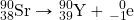Chapter 15. Nuclear Chemistry

# End-of-Chapter Material

1. Given that many elements are metals, suggest why it would be unsafe to have radioactive materials in contact with acids.
2. Many alpha-emitting radioactive substances are relatively safe to handle, but inhaling radioactive dust can be very dangerous. Why?
3. Uranium can be separated from its daughter isotope thorium by dissolving a sample in acid and adding sodium iodide, which precipitates thorium(III) iodide:

Th3+(aq) + 3I(aq) → ThI3(s)

If 0.567 g of Th3+ were dissolved in solution, how many millilitres of 0.500 M NaI(aq) would have to be added to precipitate all the thorium?

4. Thorium oxide can be dissolved in acidic solution:

ThO2(s) + 4H+ → Th4+(aq) + 2H2O(ℓ)

How many millilitres of 1.55 M HCl(aq) are needed to dissolve 10.65 g of ThO2?

5. Radioactive strontium is dangerous because it can chemically replace calcium in the human body. The bones are particularly susceptible to radiation damage. Write the nuclear equation for the beta emission of strontium-90.
6. Write the nuclear equation for the beta emission of iodine-131, the isotope used to diagnose and treat thyroid problems.
7. A common uranium compound is uranyl nitrate hexahydrate [UO2(NO3)2 ⋅ 6H2O]. What is the formula mass of this compound?
8. Plutonium forms three oxides: PuO, PuO2, and Pu2O3. What are the formula masses of these three compounds?
9. A banana contains 600 mg of potassium, 0.0117% of which is radioactive potassium-40. If 1 g of potassium-40 has an activity of 2.626 × 105 Bq, what is the activity of a banana?
10. Smoke detectors typically contain about 0.25 mg of americium-241 as part of the smoke detection mechanism. If the activity of 1 g of americium-241 is 1.26 × 1011 Bq, what is the activity of americium-241 in the smoke detector?
11. Uranium hexafluoride (UF6) reacts with water to make uranyl fluoride (UO2F2) and HF. Balance the following reaction:

UF6 + H2O → UO2F2 + HF

12. The cyclopentadienyl anion (C5H5) is an organic ion that can make ionic compounds with positive ions of radioactive elements, such as Np3+. Balance the following reaction:

NpCl3 + Be(C5H5)2 → Np(C5H5)3 + BeCl2

13. If the half-life of hydrogen-3 is 12.3 y, how much time does it take for 99.0% of a sample of hydrogen-3 to decay?
14. If the half-life of carbon-14 is 5,730 y, how long does it take for 10.0% of a sample of carbon-14 to decay?
15. Although bismuth is generally considered stable, its only natural isotope, bismuth-209, is estimated to have a half-life of 1.9 × 1019 y. If the universe is estimated to have a lifetime of 1.38 × 1010 y, what percentage of bismuth-209 has decayed over the lifetime of the universe? (Hint: Be prepared to use a lot of decimal places.)
16. The most common isotope of uranium (uranium-238) has a half-life of 4.5 × 109 y. If the universe is estimated to have a lifetime of 1.38 × 1010 y, what percentage of uranium-238 has decayed over the lifetime of the universe?
17. Refer to Table 15.1 “Average Annual Radiation Exposure (Approximate)” and separate the sources of radioactive exposure into voluntary and involuntary sources. What percentage of radioactive exposure is involuntary?
18. With reference to Table 15.1 and Exercise 17, suggest ways that a practical person can minimize exposure to radioactivity.

1. Acids can dissolve many metals; a spilled acid can lead to contamination.
1. 14.7 mL
1.1. 502.15 g/mol